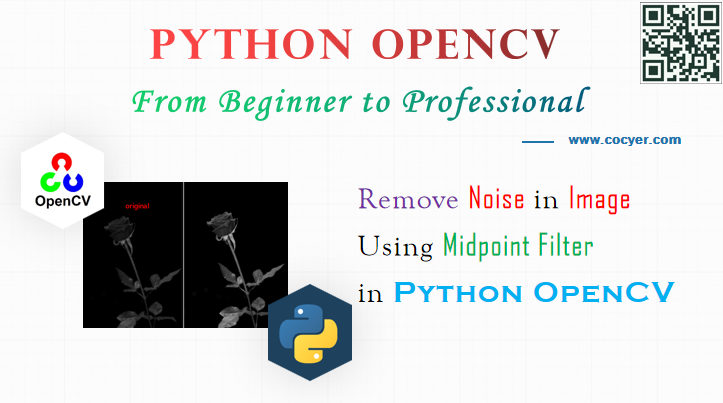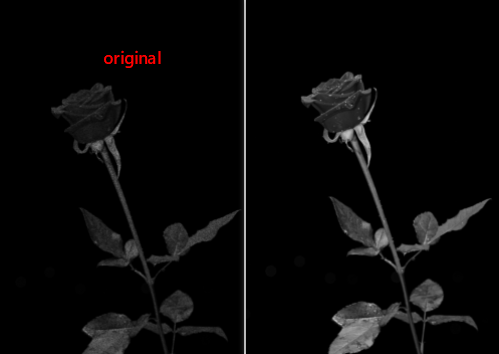# Python OpenCV: Remove Noise in Image Using Midpoint Filter

In this tutorial, we will introduce how to remove image noise using midpoint filter in python opencv. You can implement this function step by step.1.Open an image with noise

```import numpy as np
import cv2

2.Generate midpoint filter kernel

```from scipy.ndimage import maximum_filter, minimum_filter
def midpoint(img):
maxf = maximum_filter(img, (3, 3))
minf = minimum_filter(img, (3, 3))
midpoint = (maxf + minf) / 2
cv2.imshow(midpoint)```

3.Implement midpoint filter to remove noise

`midpoint(img_src)`

Run this code, you may get this image: